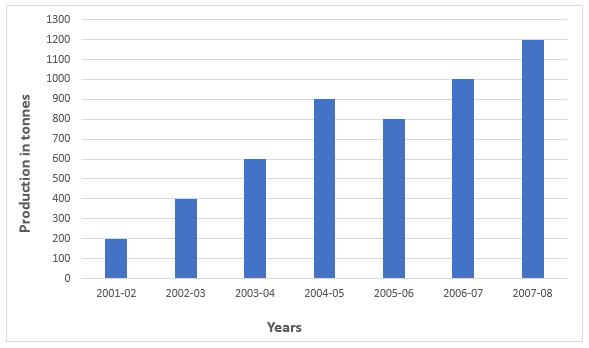## SSC MTS Quant Test 10

Instructions

Study the chart carefully and answer the questions.
The chart shows production of an item (in tonnes) during certain yearsQ 1

The production from 2003 - 04 to 2007 - 08 increased by

Q 2

The production in 2006-07 in comparison to the production in 2002-03 increased by

Q 3

The production decreased from 2004-05 to 2005-06 by ?

Q 4

The year in which production increased the lowest as compared to the previous year is

Instructions

For the following questions answer them individually

Q 5

Find the value of $$\sqrt{(x^2+y^2+2)(x+y-32)}\div \sqrt{xy^3 2^2}$$ when $$x=+1,y=-3$$